## Each of the functions below model the monetary value of an item. Which is the only that has a growth factor showing the item is

Question

Each of the functions below model the monetary value of an item. Which is the only

that has a growth factor showing the item is increasing in value?

O a(x) = 90(3/4)^x

B(x) = 215(5/4)^x

c(x) = 73(0.98)^x

d(x) = 12(0.24)^x

in progress 0
6 months 2021-08-09T14:31:36+00:00 1 Answers 3 views 0

The correct answer is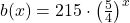.

Step-by-step explanation:

We must remember that each option represents a geometrical progression, whose model is represented by: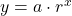Where: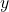– Dependent variable, dimensionless.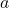– Initial value, dimensionless.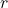– Common ration, dimensionless.

A geometric function is decreasing and monotone when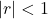, stable when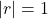and increasing and divergent when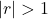.

The equationis the only one that is increasing in value, as notice that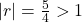. Therefore, the correct answer is B.